# How to Convert Uppercase to Lowercase Except the First Letter in Excel

In excel, words can be entered in uppercase, lowercase or mixed. If we want to convert all uppercase to lowercase (except the first letter in some situations), we can use formula with some letter convert related functions to convert them to proper case. This article will introduce you some methods to convert letters from uppercase to lowercase, it can help you to solve your problem.

Precondition:

Prepare below table. To convert uppercase to lowercase for worlds or sentence, actually there are two forms after converting. We can convert uppercase to lowercase but keep the first uppercase for each word; in another way, we can convert uppercase to lowercase but only keep the first uppercase for the first word in the sentence.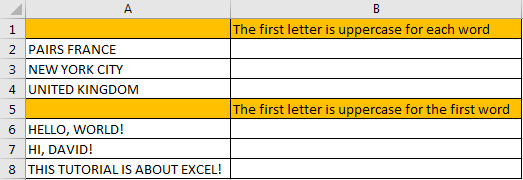## Part 1: Convert Uppercase to Lowercase Except the First Letter for Each Word

Step 1: In B2 enter the formula =PROPER(A2).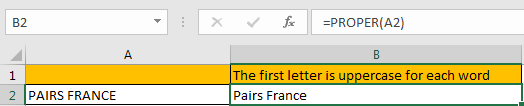For PROPER function, it converts a text or string to proper case, actually it converts the first letter in each word to uppercase, but keeps other letters in lowercase. So, no matter letters are entered in uppercase or lowercase or mixed, it will be finally displayed with the first letter in uppercase with the other letters in lowercase after applying this function.

Step 2: Drag the fill handle down to fill other cells. Verify that uppercase is converted to lowercase properly.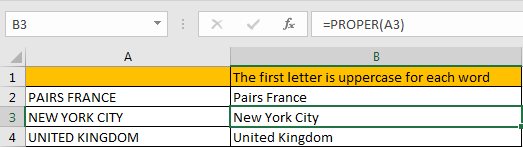## Part 2: Convert Uppercase to Lowercase Except the First Letter for the First Word

Step 1: In B6 enter the formula =UPPER(LEFT(A6,1))&LOWER(RIGHT(A6,LEN(A6)-1)).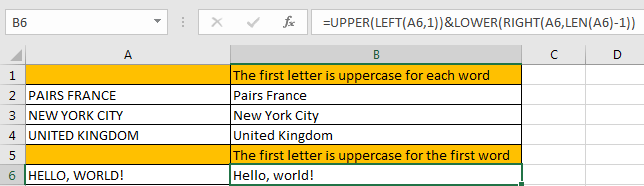This formula is combined with UPPER and LOWER two functions. It is easy to understand. For UPPER function, keep the first letter from left displaying in uppercase; for LOWER function, keep other letters except the first letter from left displaying in lowercase.

Step 2: Drag the fill handle down to fill other cells. Verify that uppercase is converted to lowercase properly.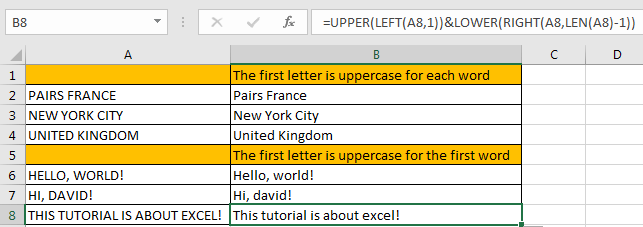### Related Functions

• Excel UPPER function
The Excel UPPER function converts all characters in text string to uppercase.The UPPER function is a build-in function in Microsoft Excel and it is categorized as a Text Function.The syntax of the UPPER function is as below:= UPPER (text)…
• Excel LOWER function
The Excel LOWER function converts all characters in a text string to lowercase.The syntax of the LOWER function is as below:= LOWER  (text)…
• Excel LEFT function
The Excel LEFT function returns a substring (a specified number of the characters) from a text string, starting from the leftmost character.The LEFT function is a build-in function in Microsoft Excel and it is categorized as a Text Function.The syntax of the LEFT function is as below:= LEFT(text,[num_chars])…
• Excel RIGHT function
The Excel RIGHT function returns a substring (a specified number of the characters) from a text string, starting from the rightmost character.The syntax of the RIGHT function is as below:= RIGHT (text,[num_chars])…
• Excel LEN function
The Excel LEN function returns the length of a text string (the number of characters in a text string).The syntax of the LEN function is as below:= LEN(text)…

Related Posts

How To Convert Text to Upper Cases(Using VBA) in Excel

This post will show you how to switch from lower case to upper case in Excel. and I am going to show you two different ways of converting text to upper cases using formula or VBA macro in Excel 2013,Excel ...

How To Force Text String to Upper,Lower, or Proper Case in Excel

This post will guide you how to force test strings to uppercase or lowercase or Proper cases through an Excel formula or data validation in your Excel 2013/2016. How to convert text to upper, lower, or proper case in Excel. ...

How to Remove the First/Last Word from Text string in Cell

This post will guide you how to remove the first and the last word from a text string in cells in Excel. How do I use a formula to remove first and last word of a text string in Excel. ...

How to Remove Prefix and Suffix in Given Cells in Excel

This post will guide you how to remove suffix form a range of cells in Excel. How do I remove prefix from text string in cells with a formula in Excel. Remove Prefix of Cells Remove Suffix of Cells Assuming ...

How to Check If a Cell is Uppercase, Lowercases or Capitalize in Excel

This post will guide you how to check if a cell is uppercase in Excel 2013/2016. How do I identify all letters in cells are lowercases or capitalize in Excel. How to change letters to uppercase, lowercases or capitalize first ...

This post will guide you how to extract email address from a text string in Excel. How do I use a formula to extract email address in Excel. How to extract email address from text string with VBA Macro in ...

Convert Scientific Notation to Text or a Number in Excel

This post will guide you how to convert scientific notation to text or a number in Excel. How do I convert excel scientific to text with a formula in Excel. Convert Scientific Notation to Text or a Number in Excel ...

Extract First or Last N Characters

This post will guide you how to extract the first or last n characters from a text string in one cell in Excel. How do I get the first or last N characters from a string using an Excel formula. ...

Insert The File Path and Filename into Cell

This post will guide you how to insert the file path and filename into a cell in Excel. Or how to add a file path only in a specified cell with a formula in Excel. Or how to add the ...

Extract Part of Text String

This post will guide you how to extract part of text string from a cell in Excel. How do I extract a word from a text string in Excel. How to extract a substring from a text string in a ...

Sidebar